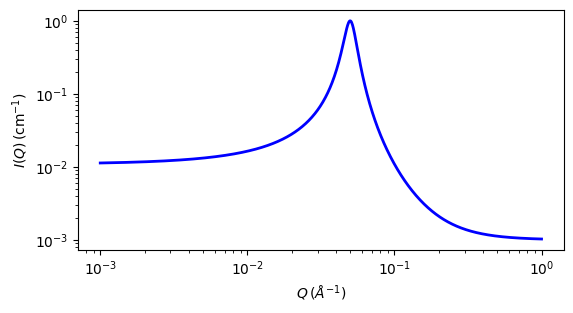# peak_lorentz

A Lorentzian peak on a flat background

Parameter

Description

Units

Default value

scale

Scale factor or Volume fraction

None

1

background

Source background

cm-1

0.001

peak_pos

Peak postion in q

-1

0.05

peak_hwhm

HWHM of peak

-1

0.005

The returned value is scaled to units of cm-1 sr-1, absolute scale.

This model describes a Lorentzian shaped peak on a flat background.

Definition

The scattering intensity $$I(q)$$ is calculated as

$I(q) = \frac{scale}{\bigl(1+\bigl(\frac{q-q_0}{B}\bigr)^2\bigr)} + background$

with the peak having height of $$I_0$$ centered at $$q_0$$ and having a HWHM (half-width half-maximum) of B.

For 2D data the scattering intensity is calculated in the same way as 1D, where the $$q$$ vector is defined as

$q = \sqrt{q_x^2 + q_y^2}$Fig. 113 1D plot corresponding to the default parameters of the model.

Source

peak_lorentz.py

References

None.

Authorship and Verification

• Author: